JEE  >  Revision Notes: Some Basic Concepts in Chemistry

# Revision Notes: Some Basic Concepts in Chemistry Notes | Study Mock Test Series for JEE Main & Advanced - JEE

## Document Description: Revision Notes: Some Basic Concepts in Chemistry for JEE 2022 is part of Physical Chemistry for Mock Test Series for JEE Main & Advanced preparation. The notes and questions for Revision Notes: Some Basic Concepts in Chemistry have been prepared according to the JEE exam syllabus. Information about Revision Notes: Some Basic Concepts in Chemistry covers topics like What is a Matter?, Classification of Matter, What is Pure Substance?, What is a Mixture?, Physical Quantities &amp; their Measurement, What is Dalton’s Atomic Theory?, Precision and Accuracy&nbsp;, Significant Figures: Rules, Scientific Notation, Laws of Chemical Combination, Atomic and Molecular Masses, Mole Concept, Empirical Formula and Molecular Formula, Concentration of the Solutions and Revision Notes: Some Basic Concepts in Chemistry Example, for JEE 2022 Exam. Find important definitions, questions, notes, meanings, examples, exercises and tests below for Revision Notes: Some Basic Concepts in Chemistry.

Introduction of Revision Notes: Some Basic Concepts in Chemistry in English is available as part of our Mock Test Series for JEE Main & Advanced for JEE & Revision Notes: Some Basic Concepts in Chemistry in Hindi for Mock Test Series for JEE Main & Advanced course. Download more important topics related with Physical Chemistry, notes, lectures and mock test series for JEE Exam by signing up for free. JEE: Revision Notes: Some Basic Concepts in Chemistry Notes | Study Mock Test Series for JEE Main & Advanced - JEE
 Table of contents What is a Matter? Classification of Matter What is Pure Substance? What is a Mixture? Physical Quantities & their Measurement What is Dalton’s Atomic Theory? Precision and Accuracy Significant Figures: Rules Scientific Notation Laws of Chemical Combination Atomic and Molecular Masses Mole Concept Empirical Formula and Molecular Formula Concentration of the Solutions
 1 Crore+ students have signed up on EduRev. Have you?

What is a Matter?

Anything that has mass and occupies space is called matter.

The following are the characteristics of the matter:

• Particles have space between them
• Particles are continuously moving
• Particles attract each other

Classification of Matter

Based on the chemical composition of various substances matter is classified as Pure Substances & Mixtures.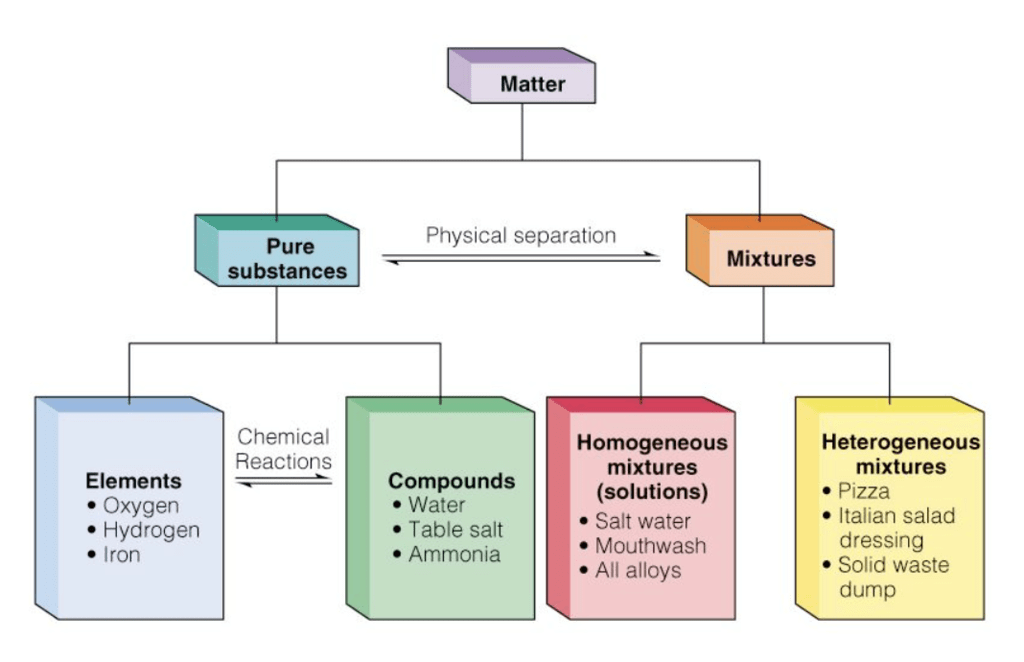What is Pure Substance?

Pure substances are substances that are made up of only one kind of particles and has a fixed or constant structure.

Types of Pure Substances

Pure substances are further classified as elements and compounds.

➢ Elements

• It is the simplest form of the matter.
• The smallest unit of an element is known as an atom.
• The total number of the known elements is 118 out of which 98 elements occur naturally and 20 are formed by artificial transmutation.
• Examples: Na, K, Mg. Al, Si, P, C, F, Br etc.

➢ Compound

• It is a non-elemental pure compound.
• Formed by the chemical combination of two or more atoms of different elements in a fixed ratio.
• Examples: H2O, CO2, C6H12O6 etc.

Characteristics & Properties Of Pure Substances

• Pure substances are mostly homogeneous in nature containing only one type of atoms or molecules.
• These substances mainly have a constant or uniform composition throughout.
• The substances have fixed boiling and melting points.
• A pure substance usually participates in a chemical reaction to form predictable products.

What is a Mixture?

In chemistry, when two or more substances mix with each other without participating in a chemical change, the resulting substance is called a Mixture.

Types of Mixtures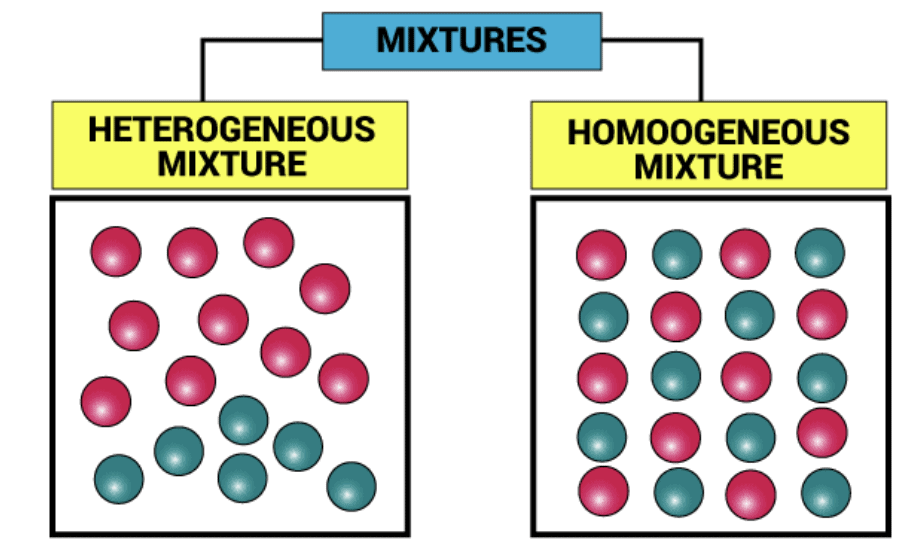➢ Homogeneous mixtures are those whose composition for each part remains constant.
Example: Aqueous and gaseous solution.

➢ Heterogeneous mixtures are those whose composition may vary for each and every part.

Example: Soil and concrete mixtures.

Question for Revision Notes: Some Basic Concepts in Chemistry
Try yourself:A ________ is made up of two or more pure substances which may be in any ratio.

Physical Quantities & their Measurement

Fundamental Units

• These units can neither be derived from one another nor can be further resolved into any other units. Seven fundamental units of the S.I. system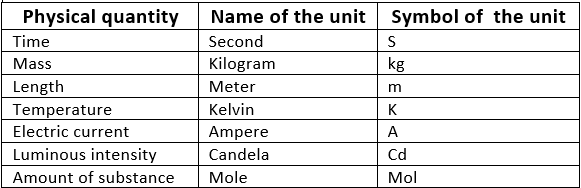Derived Units

• These units are the function of more than one fundamental unit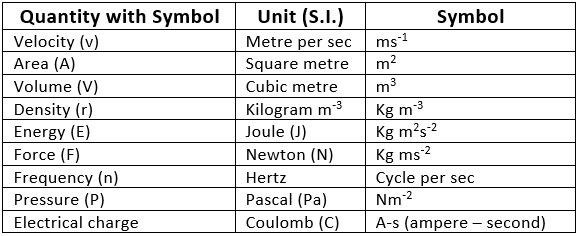Measurement of Temperature

➢ Three scales of temperature

• Kelvin scale (K)
• Degree Celsius scale (oC)
• Degree Fahrenheit  scale (oF)

➢ Relations between the scales:

• oF = 9/5(oC) + 32
• K = oC + 273

0 K temperatures are called absolute zero.

What is Dalton’s Atomic Theory?

Dalton's atomic theory proposed that all matter was composed of atoms, indivisible and indestructible building blocks. While all atoms of an element were identical, different elements had atoms of differing size and mass.

• Every matter consists of indivisible atoms.
• Atoms can neither be created nor destroyed.
• Atoms of a given element are identical in  properties
• Atoms of different elements differ in properties.
• Atoms of different elements combine in a fixed ratio to form a molecule of a compound.

Question for Revision Notes: Some Basic Concepts in Chemistry
Try yourself:According to Dalton’s Atomic Theory, matter consists of indivisible _______

Precision and Accuracy

• Precision: Closeness of outcomes of different measurements taken for the same quantity.
• Accuracy: Agreement  of experimental value to the true value

Significant Figures: Rules

• All non-zero digits are significant.
• Zeroes preceding the first non-zero digit are not significant.
• Zeroes between two non-zero digits are significant.
• Zeroes at the end of a number are significant when they are on the right side of the decimal point.
• Counting numbers of objects have infinite significant figures.

Question for Revision Notes: Some Basic Concepts in Chemistry
Try yourself:How many significant figures does 63180 have?

Scientific Notation

Numbers are represented in N × 10n form.
Where,

• N = Digit term
• n = exponent having positive or negative value.

Examples:
12540000 = 1.254 × 107
0.00456 = 4.56 ×10-3

Mathematical Operations of Scientific Notation

➢ Multiplication and Division

• Follow the same rules which are for exponential number.
Example: (7.0×103)×(8.0×10-7) =  (7.0×8.0)×(10[3 + (-7)]) = 56.0×10-4
• Result cannot have more digits to the rite of decimal point than either of the original numbers.
(7.0×103)/(8.0×10-7) = (7.0/8.0)×(10[3 - (-7)]) =  0.875×1010  =  0.9×1010

• Numbers are written in such a way that they have the same exponent and after that coefficients are added or subtracted.
(5×103) + (8×105) = (5×103) + (800×103) = (5+800)×103  = 805×103
• The result must be reported with no more significant figures as there in the original number with few significant figures.

➢ Rules for limiting the result of mathematical operations:

• If the rightmost digit to be removed is more than 5, the preceding number is increased by one.
• If the rightmost digit to be removed is less than 5, the preceding number is not changed.
• If the rightmost digit to be removed is 5, then the preceding number is not changed if it is an even number but is increased by one if it is an odd number.

➢ Dimensional Analysis

• This is based on the fact that the ratio of each fundamental quantity in one unit with their equivalent quantity in another unit is equal to one.
• Derived unit first expressed in dimension and each fundamental quantities like mass length time are converted in another system of the desired unit to work out the conversion factor
• Original Quantity × Conversion factor = Equivalent Quantity
(In former unit)                                  (In final Unit)
Example: (1 kg/2.205 pound) = 1=(1kg/1000gm)
So 1 kg = 2.205 pound = 1000 gm

Laws of Chemical Combination

Law of conservation of mass

“For any chemical change total mass of active reactants are always equal to the mass of the product  formed”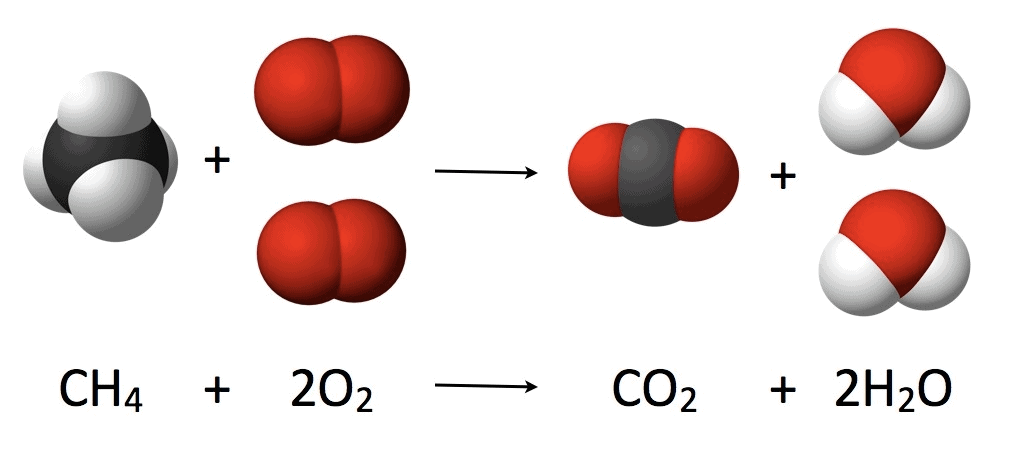Law of constant proportions

A chemical compound always contains same elements in definite proportion by mass and it does not depend on the source of compound.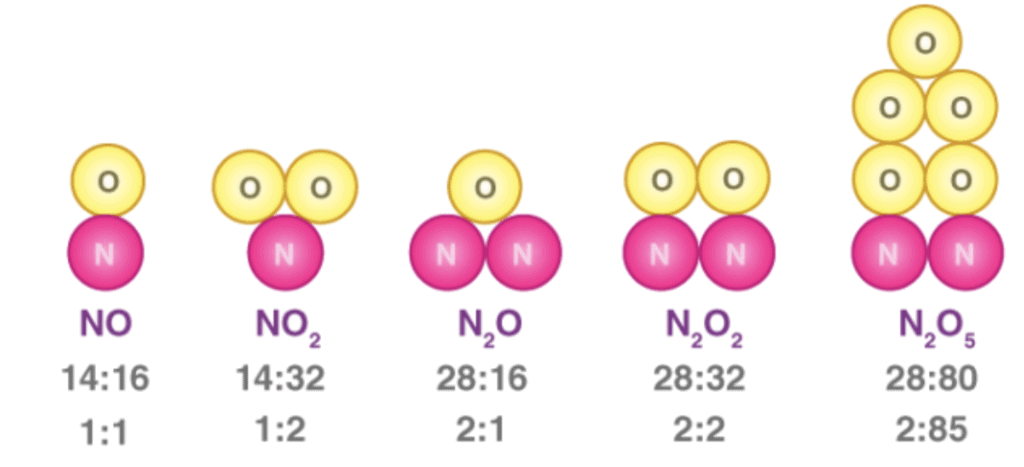Law of multiple proportions

When two elements combine to form two or more than two different compounds then the different masses  of one element B which combine with fixed mass of the other element bear a simple ratio to one another.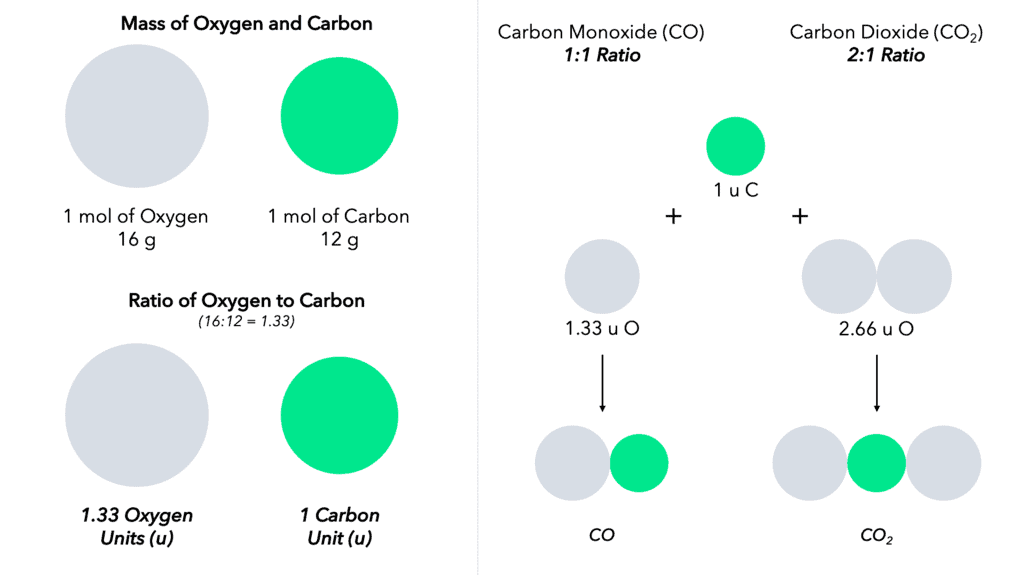Law of reciprocal proportion

If two elements B and C react with the same mass of a third element (A), the ratio in which they do so will be the same or simple multiple if B and C reacts with each other.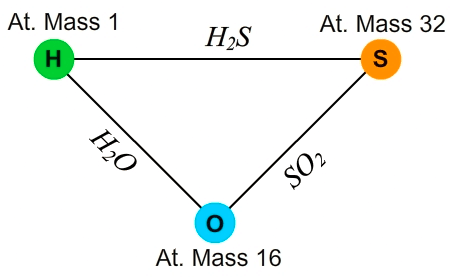Gay Lussac’s law of combining volumes

At given temperature and pressure the volumes of all gaseous reactants and products bear a simple whole number ratio to each other.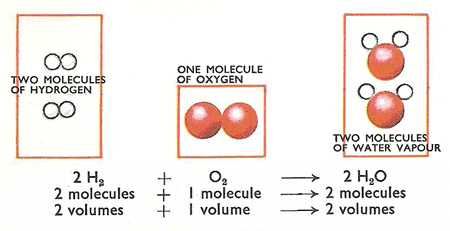Question for Revision Notes: Some Basic Concepts in Chemistry
Try yourself:What did Dalton propose?

Atomic and Molecular Masses

➢ Atomic Mass

• Mass of an atom.
• Reported in the atomic mass unit “amu” or unified mass “u”
• One atomic mass unit i.e. amu, is the mass exactly equal to one-twelfth the mass of one carbon-12 atom.

➢ Molecular Mass

• Mass of a molecule of the covalent compound.
• It is equal to the sum of atomic masses of all the elements present in the molecule.

➢ Formula Unit Mass

• Mass of a molecule of an ionic compound
• It is also equal to the sum of atomic masses of all the elements present in the molecule

Mole Concept

➢ Mole

• Unit of the amount of substance.
• One mole amount of substance that contains as many particles or entities as there are atoms in exactly 12 g of the 12C isotope.

➢ Molar mass

• Mass of one mole of a substance in gram
• Molar mass in gram in numerically equal to atomic/molecular/formula mass in amu or u.

➢ Percentage composition

Mass percentage of an element in a compound = (Mass of that element in the compound/Molecular mass of the compound)×100

➢ Percentage yield

• It is the ratio of the actual yield of the reaction to the theoretical yield multiplied by 100.
• % yield = (Actual yield /Theoretical yield)×100

Empirical Formula and Molecular Formula

➢ Molecular Formula

Represents the actual number of each individual atom in any molecule is known as the molecular formula.

➢ Empirical Formula

Expresses the smallest whole number ratio of the constituent atom within the molecule.

Molecular formula = (Empirical formula)n
Molecular weight = n × Empirical weight
also,
Molecular weight = 2 × Vapour density

➢ Limiting Reagent

• The reactant which is totally consumed during the course of reaction and when it is consumed reaction stops.
• For a balanced reaction reaction:
A +B →  C + D
B would be a limiting reagent if n/ nB>nB/nA
Similarly, A is a limiting reagent if nA / nB<nB/nA

Concentration of the Solutions

➢ Mass by Mass Percentage

• Amount of solute in gram present per 100 gm of the solution.
• Mass percentage of solute = [(Mass of solute)/(Mass of solution)] x100
• Mass by Volume Percentage
• Amount solute in gram present per 100 mL of the solution.

➢ Volume by Volume Percentage

• The volume of solute per 100 mL of the solution
• Volume by volume percentage of solute = [(Volume of solute)/(volume of solution)] x100

➢ Parts per million (ppm)

• The amount of solute in gram per million (106) gram of the solution.
• ppm = [(mass of solute/mass of solution)]x 106

➢ Mole fraction

• Ratio of the moles of one component of the solution to the total number of moles of solution.
• Total mole fraction of all the components of a solution is equal to 1.
• For binary solutions having two components A and B

Mole fraction of A
XA =  (nA)/(nA+nB)]
Mole fraction of B
X=  (nB)/(nA+nB)]
or  XB = 1- XA

➢ Molarity (M)

• A number of moles of solute per 1000 mL of the solution.
• M = (Number of moles of solute)/(Volume of solution in L)

➢ Molality (m)

• The number of moles of solute per 1000 gram of the solvent.
• m = (Number of moles of solute)/(Weight of solvent in kg)

Question for Revision Notes: Some Basic Concepts in Chemistry
Try yourself:Which of the following cannot be a molecular formula for an empirical formula HO?

The document Revision Notes: Some Basic Concepts in Chemistry Notes | Study Mock Test Series for JEE Main & Advanced - JEE is a part of the JEE Course Mock Test Series for JEE Main & Advanced.
All you need of JEE at this link: JEE

## Mock Test Series for JEE Main & Advanced

2 videos|324 docs|160 tests
 Use Code STAYHOME200 and get INR 200 additional OFF

## Mock Test Series for JEE Main & Advanced

2 videos|324 docs|160 tests

Track your progress, build streaks, highlight & save important lessons and more!

,

,

,

,

,

,

,

,

,

,

,

,

,

,

,

,

,

,

,

,

,

;